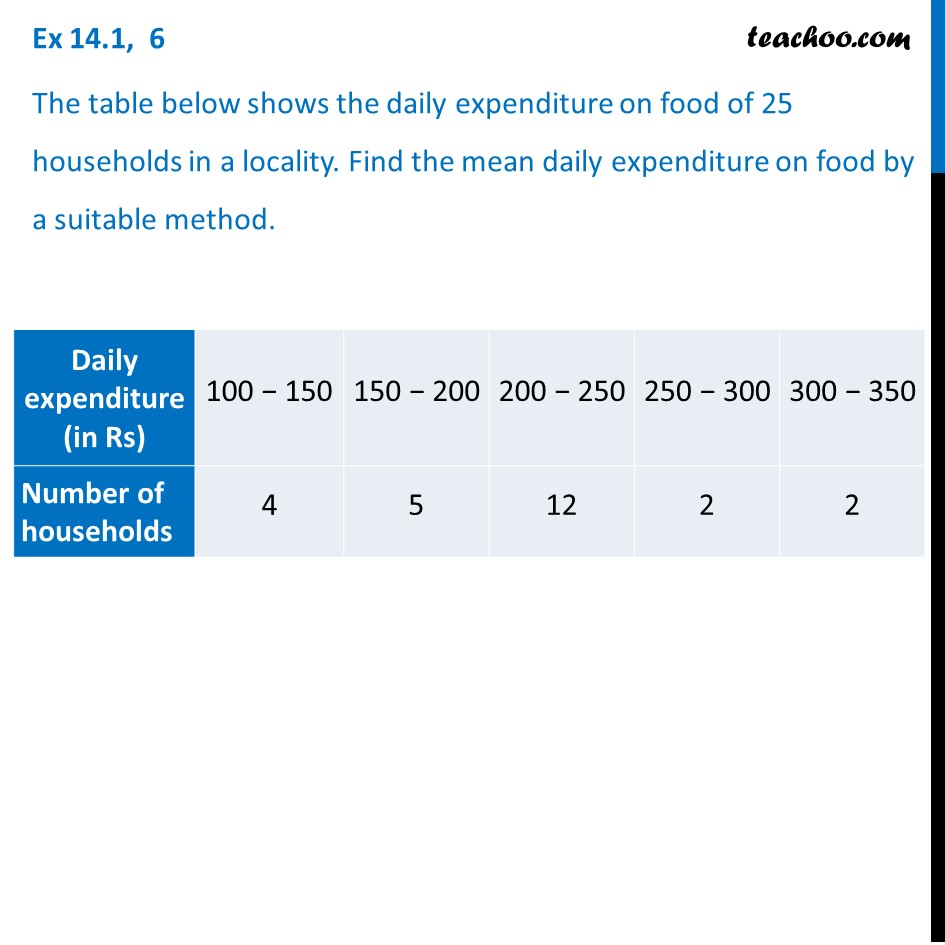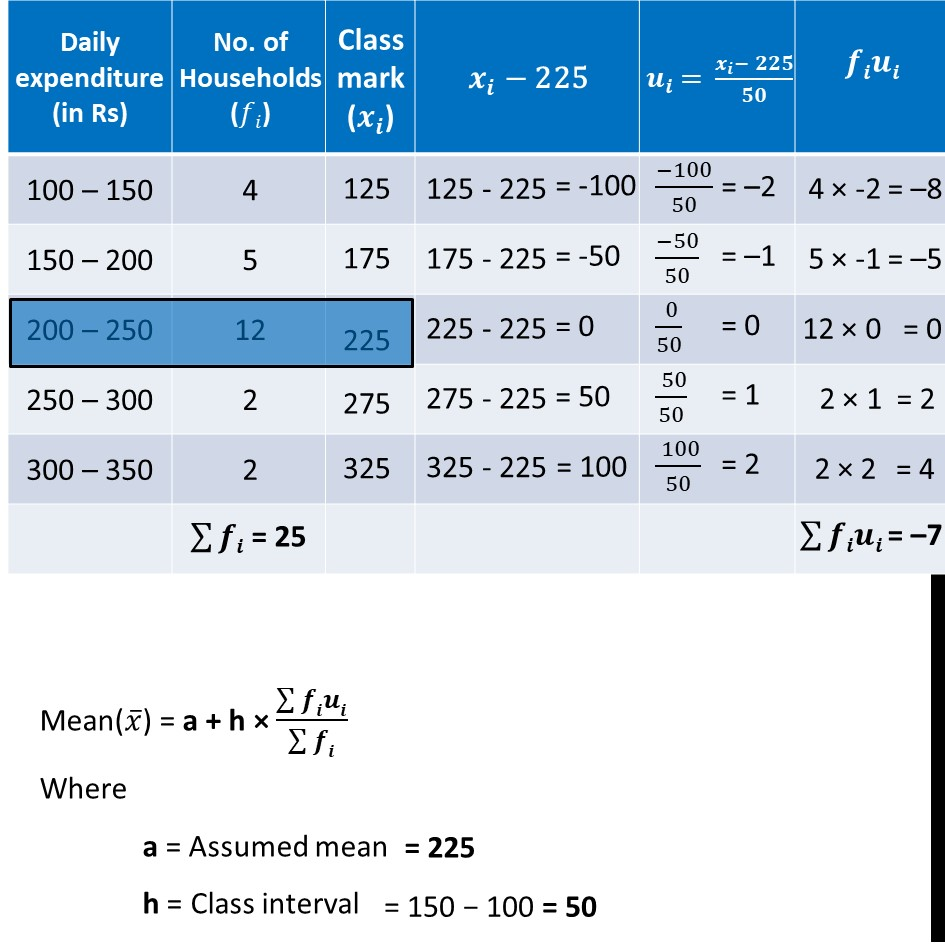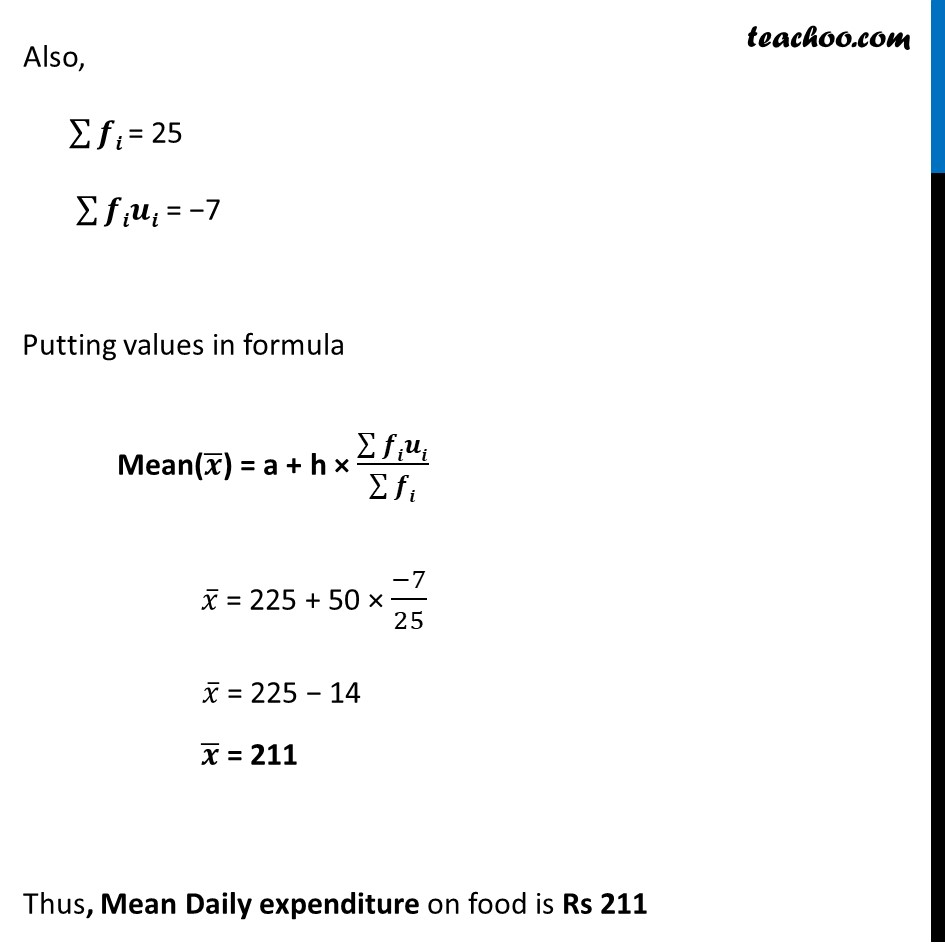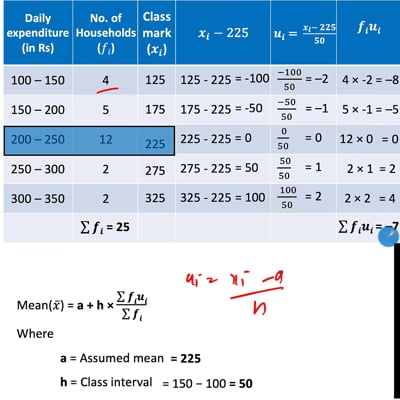Mean

Chapter 14 Class 10 Statistics
Concept wiseThis video is only available for Teachoo black users

Introducing your new favourite teacher - Teachoo Black, at only ₹83 per month

### Transcript

Ex 14.1, 6 The table below shows the daily expenditure on food of 25 households in a locality. Find the mean daily expenditure on food by a suitable method. Mean(𝑥 ̅) = a + h × (∑▒𝒇𝒊𝒖𝒊)/(∑▒𝒇𝒊) Where a = Assumed mean h = Class interval = 225 = 150 − 100 = 50 Also, ∑▒𝒇𝒊 = 25 ∑▒𝒇𝒊𝒖𝒊 = −7 Putting values in formula Mean(𝒙 ̅) = a + h × (∑▒𝒇𝒊𝒖𝒊)/(∑▒𝒇𝒊) 𝑥 ̅ = 225 + 50 × (−7)/25 𝑥 ̅ = 225 − 14 𝒙 ̅ = 211 Thus, Mean Daily expenditure on food is Rs 211